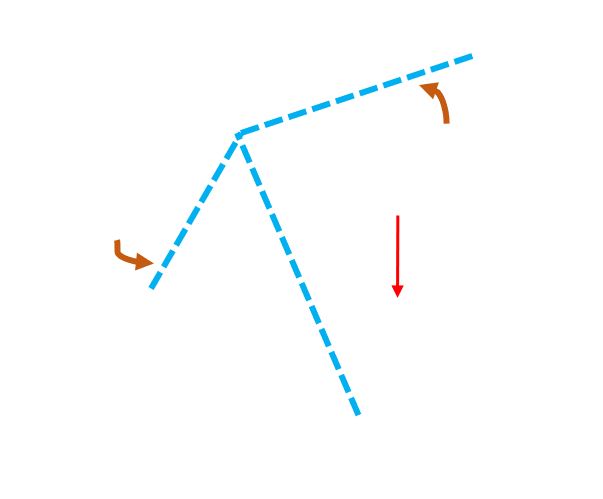# Michael Wrona's Blog

## Engineering, coding, tech, and other cool projects!

One of the most important components in control systems for airplanes, fighter jets, quadcopters, and rockets is the AHRS, or Attitude and Heading Reference System. As you can guess, the AHRS tells a system its current attitude orientation and heading. Attitude measurements are typically given as roll and pitch angles, and heading is relative to either magnetic or true north. In order to control a system as complex (and expensive) as airplanes and rockets, a very well-engineered and robust AHRS system is required.

An AHRS consists of three different sensors: a three-axis accelerometer, a three-axis gyroscope, and a three-axis magnetometer. The accelerometer measures accelerations, the gyroscope measures angular rotation rates, and the mangetometer measures magnetic fields (including earth’s magnetic field). In addition to accelerations due to movement, the accelerometeter measures gravitational, linear, coriolis, and centripetal accelerations. Since we know gravity’s direction is straight down, and its mangitude is 9.81 m/s/s, we can use this knowledge to begin computing orientation!

## Important Notes

• We must assume the accelerometer remains stationary, therfore only measuring acceleration due to gravity. If the sensor moves/accelerates, the method outlined in this article will no longer be valid. It should still work for most applications, accept dynamics ones.
• The accelerometer can only measure roll and pitch angles, not heading. Gravity is down. No matter the orientation of the accelerometer, it will never be able to measure heading. To put this simply, you can’t measure heading with an accelerometer because gravity doesn’t depend on heading.

## DerivationVisualization of angles, where (X, Y, Z) is the level frame, and (X’, Y’, Z’) is the tilted frame.

If we express the gravitational acceleration vector in a level frame, with the +z-axis in the same direction as gravity, it would be:

$$\vec{g}_L = \begin{bmatrix} 0 & 0 & g \end{bmatrix}$$

Where $g = 9.81m/s^2$ and L refers to the level frame (no tilt, completely flat). The accelerometer measurement in a tilted frame will be:

$$\vec{a}_T = \begin{bmatrix} a_x & a_y & a_z \end{bmatrix}$$

Where T refers to the tilted frame and $a_i$ refers to the accelerometer measurement for each axis. In order to describe the orientation of our tilted frame relative to the level one, we will be using Euler angles; more specifically, the 3-2-1 Euler angle set. I included a few resources at the bottom of the page if you need to brush up on them. The 3-2-1 Euler angles are referred to as yaw ($\psi$), pitch ($\theta$), and roll ($\phi$), respectively, and are commonly used in aircraft dynamics and controls. We will use the 3-2-1 Euler angle direction cosine matrix, or DCM, to convert between the tilted frame and level frame. Since we are ignoring yaw/heading measurements, the DCM relationship between the tilted frame and level frame is:

$$\begin{pmatrix} a_x \\ a_y \\ a_z \end{pmatrix}_T = \begin{pmatrix} cos\phi & 0 & -sin\theta \\ sin\theta sin\phi & cos\phi & cos\theta sin\phi \\ sin\theta cos\phi & -sin\phi & cos\theta cos\phi \end{pmatrix} \begin{pmatrix} 0 \\ 0 \\ -g \end{pmatrix}_L$$

If we carry out the matrix-vector multiplications, we get the following equations:

$$a_x = g sin\theta$$ $$a_y = -g sin\phi cos\theta$$ $$a_z = -g cos\phi cos\theta$$

Using the first equation to solve for $\theta$, we get:

$$Pitch = \theta = arcsin \frac{a_x}{g}$$

Using the second and third equations to solve for $\phi$, and noting that $tan = \frac{sin}{cos}$, we get:

$$Roll = \phi = arctan \frac{a_y}{a_z}$$

Using the fact that $g = \sqrt{a_x^2 + a_y^2 + a_z^2}$ for a stationary sensor, we arrive at the two final expressions:

$$Pitch = \theta = arcsin \frac{a_x}{g} = arcsin \frac{a_x}{\sqrt{a_x^2 + a_y^2 + a_z^2}}$$ $$Roll = \phi = arctan \frac{a_y}{a_z}$$

$$g = 9.81 \, m/s^2$$

Using these two expressions, we can convert accelerometer measurements into roll and pitch angles!